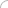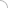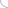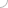# Clocks - aptitude test

Home > Aptitude test > Clocks

### 1) Find the angle between the hour hand and the minute hand of a clock when the time is 5.45.

a) 97.5°
b) 90°
c) 100°
d) 95°

Solution:

Angle traced by the hour hand in 12 hours = 360°
Angle traced by the hour hand in 5 hours 45 minutes = (360 * 23) / (12 *4) = 172.5°
Angle traced by the minute hand in 60 minutes = 360°
Angle traced by the minute hand in 45 minutes = 270°
Therefore, the angle between the hour hand and the minute hand at 5.45
= (270 – 172.5) = 97.5°

### 2) The reflex angle between the hands of a clock at 9.35 is:

a) 270°
b) 77.5°
c) 282.5°
d) 300°

Solution:

Angle traced by the hour hand in 12 hours = 360°
Angle traced by the hour hand in 9 hours 35 minutes = (360 * 115) / (12 * 12) = 287.5°
Angle traced by the minute hand in 60 minutes = 360°
Angle traced by the minute hand in 35 minutes = 210°
Therefore, the angle between the hour hand and the minute hand at 9.35
= (287.5 – 210) = 77.5°
Reflex angle = 360 – 77.5 = 282.5°

### 3) Find the number of times the hour hand and the minute hand of a clock are at right angle in a day.

a) 48
b) 24
c) 22
d) 44

Solution:

In 12 hours, the hands of a clock are at right angle 22 times.
Hence in a day they are at right angle 44 times.

### 4) A clock was set right at 2 p.m. It gains 5 seconds in 3 minutes, and it indicates 8.30 a.m. the next morning, then the true time is:

a) 8.30 a.m.
b) 7.45 a.m.
c) 8.15 a.m.
d) 7.30 a.m.

Solution:

Time elapsed from 2 p.m. to 8.30 a.m. = 18 hours 30 minutes = 1110 minutes.
Now, 3 minutes and 5 seconds of the given clock is 3 minutes of the correct clock.
Therefore, 1110 minutes of this clock is (1110 * 3) / (37/12) minutes of the correct clock.
= 1080 minutes = 18 hours of the correct clock
Hence, the correct time is 8 a.m.

 Discussion Board clock questions yes i a this am not understanding this chapter raman singh 07-25-2020 07:42 AMAptitude questions